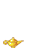Hi, I am Maomei, a PhD student in the Department of Philosophy at the University of Hong Kong (HKU) under the supervision of David McCarthy. Before coming to HKU, I got my MPhil degree under the supervision of Jiji Zhang and Simon Goldstein at Lingnan University.

I am interested in the following question: what should I do in the face of uncertainty? This is a quite general question. I hope I could make it clear in my life. For now, I am interested in the following specific questions:

1. Why Probabilism/Imprecise Probabilism (representation)?

2. How to incorporate eveidence (updating)?

3. The characterizations of different independence notions and statistical learning (esp., learning a probabilistic hypothesis).

4. The axiomatic foundations of expected utility maximizationm, maximin and minimax regret (decision rules).

5. The choice of loss functions, e.g., why are these reasonable measures of distances between probabilistic distributions?

My current research focuses on 1 and 2. To be specific, I am investigating: (i) the rationales for Probabilism/Imprecise Probabilism, and (ii) how we should update, using the decision theoretic approach (so it also relates to 4). Previously, I wrote my MPhil thesis on the accuracy approach to Probabilism,  which relates to 1 and 5.

Apart from the above, I am also interested in a variety of topics in logic, e.g., Gödel's incompleteness theorems, whether NP = P, etc. I do not know much about logic, but I would be happy if you discuss related things with me!You can contact me at mmmw@connect.hku.hk.

TOP